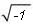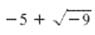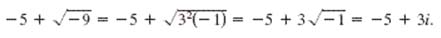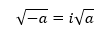The Imaginary Unit i

The Imaginary Unit i

Some quadratic equations have no real solutions.  For instance, the quadratic equation x2 + 1 = 0 has no real solution because there is no real number x that can be squared to produce -1.  To overcome this deficiency, mathematicians created an expanded system of numbers using the imaginary unit i, defined as --

i =where  i 2 =  -1.

Definition of a Complex Number

By adding real numbers to real multiples of the imaginary unit, a set of complex numbers is obtained.  Each complex number can written in the standard form a + bi

The letter a stands for the "real" part of the complex number and the number bi (where b is a real number not equal to zero) is called the imaginary part of the complex number.

Example

Write the complex number below
in standard form.Solution

-5 + 3i

Since,Principal Square Root of a Negative Number

If a is a positive number, the principal square root of the negative number -a is defined as --